# Chapter 10 Light - Reflection and Refraction

We all are familiar with word light. Light produces us the sensation of sight. During day time, sunlight falls on the objects get reflected and we are able to see the object. But at night we cannot see the object and cannot identify the colour, while lighting the bulb we are able to see the object. You have seen shadow of our own and others because light travels along a straight path. In this chapter we will understand the characteristics of light, reflection, refraction and diffraction of light, formation of an image on a plane mirror, spherical mirror & lense and their application in real life.

Download pdf of NCERT Solutions for Class Science Chapter 10 Light - Reflection and Refraction

Download pdf of NCERT Examplar with Solutions for Class Science Chapter 10 Light - Reflection and Refraction

### Exercise 1

•  Q1 Define the principal focus of a concave mirror. Ans: The ray of light  that is parallel to the principal axis of a concave mirror converges at a specific point on its principal axis after reflecting from the mirror. This point is known as the principal focus of the concave mirror. Q2 The radius of curvature of a spherical mirror is 20 cm. What is its focal length? Ans: Given, Radius of curvature, R = 20 cm Radius of curvature of a spherical mirror = 2 × Focal length (f) R = 2f    by putting the values we get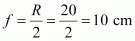The focal length of the given spherical mirror is 10 cm. Q3 Name a mirror that can give an erect and enlarged image of an object. Ans: The image formed is virtual, erect, and enlarged.When an object is placed between in the pole and the principal focus of a concave mirror. Q4 Why do we prefer a convex mirror as a rear-view mirror in vehicles? Ans: Convex mirrors are preferred as rear view mirrors because they give a virtual, erect, and diminished image of the objects when placed in front of them and  cover a wider field of view, which allows the driver to see the traffic behind him.

### Exercise 2

•  Q1 Find the focal length of a convex mirror whose radius of curvature is 32 cm. Ans: Given: Radius of curvature, R = 32 cm Radius of curvature = 2 × Focal length (f) R = 2f  On putting the values we get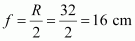Therefore, the focal length of the given convex mirror is 16 cm. Q2 A concave mirror produces three times magnified (enlarged) real image of an object placed at 10 cm in front of it. Where is the image located? Ans: Magnification produced by a spherical mirror is expressed as.Suppose, the height of the object, ho = h Then, height of the image, hI = −3h (Image formed is real)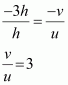Object distance, u = −10 cm v = 3 × (−10) = −30 cm Here, the negative sign indicates that an inverted image is formed at a distance of 30 cm in front of the given concave mirror.

### Exercise 3

•  Q1 A ray of light travelling in air enters obliquely into water. Does the light ray bend towards the normal or away from the normal? Why? Ans: A ray of light when it travels from air to water it bends towards the normal. When a ray of light travels from an optically rarer medium to an optically denser medium, it gets bent towards the normal.As water is  more optically denser than air, a ray of light travelling from air into the water will bend towards the normal. Q2 Light enters from air to glass having refractive index 1.50. What is the speed of light in the glass? The speed of light in vacuum is 3 × 108 m s–1. Ans: Refractive index of a medium nm is expressed as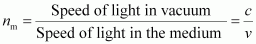Speed of light in vacuum, c = 3 × 108 m s−1 Refractive index of glass, ng = 1.50 So , Speed of light in the glass,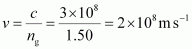Q3 Find out, from Table 10.3, the medium having highest optical density. Also find the medium with lowest optical density. Material medium Refractive index Material medium Refractive index Air 1.0003 Canada Balsam 1.53 Ice 1.31     Water 1.33 Rock salt 1.54 Alcohol 1.36     Kerosene 1.44 Carbon disulphide 1.63 Fused quartz 1.46     Turpentine oil 1.47 Ruby 1.71 Benzene 1.50 Sapphire 1.77 Crown glass 1.52 Diamond 2.42              Table 10.3 Absolute refractive index of some material media Ans: From the above provided information the object having, Highest optical density is Diamond and Lowest optical density  is Air.Optical density of a medium is directly related with the refractive index of that medium. A medium which has the highest refractive index will have the highest optical density and vice-versa. Q4 You are given kerosene, turpentine and water. In which of these does the light travel fastest? Use the information given in Table 10.3. Material medium Refractive index Material medium Refractive index Air 1.0003 Canada Balsam 1.53 Ice 1.31     Water 1.33 Rock salt 1.54 Alcohol 1.36     Kerosene 1.44 Carbon disulphide 1.63 Fused quartz 1.46     Turpentine oil 1.47 Ruby 1.71 Benzene 1.50 Sapphire 1.77 Crown glass 1.52 Diamond 2.42 Ans: Speed of light in a medium is given by the relation for refractive index (nm). The relation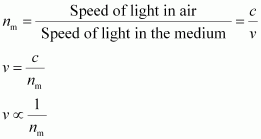It can conclude from the relation that light will travel the slowest in the material which has the highest refractive index and travel the fastest in the material which has the lowest refractive index. Therefore, light travels the fastest in water. Q5 The refractive index of diamond is 2.42. What is the meaning of this statement? Ans: The refractive index of diamond is 2.42. This means  that the speed of light in diamond will reduce by a factor 2.42 compared to its speed in air. Refractive index of a medium nm is related to the speed of light in that medium v by the relation: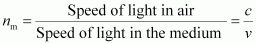Where, c is the speed of light in vacuum/air.

### Exercise 4

•  Q1 Define 1 dioptre of power of a lens. Ans: 1 dioptre is defined as the power of a lens of focal length 1 metre. ∴1 D = 1 m-1 Power of lens is defined as the reciprocal of its focal length. Where P is the power of a lens of focal length f  in metres, then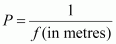The S.I. unit of power of a lens is Dioptre. It is denoted by D. Q2 A convex lens forms a real and inverted image of a needle at a distance of 50 cm from it. Where is the needle placed in front of the convex lens if the image is equal to the size of the object? Also, find the power of the lens. Ans: The image formed is inverted and of the same size as the object. When an object is placed at the centre of curvature, 2F1, of a convex lens, its image is formed at the centre of curvature, 2F2, on the other side of the lens.Given, The image of the needle is formed at a distance of 50 cm from the convex lens. Hence, the needle is placed in front of the lens at a distance of 50 cm. Object distance, u = −50 cm Image distance, v = 50 cm Focal length = f Putting values to the lens formula,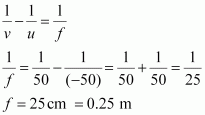Hence, the power of the given lens is +4 D. Q3 Find the power of a concave lens of focal length 2 m. Ans: Given, Focal length of concave lens, f = - 2 m Power of a lens,a negative sign is due to the divergent nature of the concave lens. Hence, the power of the given concave lens is −0.5 D.